### ISTEXT Function – Examples in Excel, VBA, Google Sheets

This tutorial demonstrates how to use the Excel ISTEXT Function in Excel to test if a cell is text.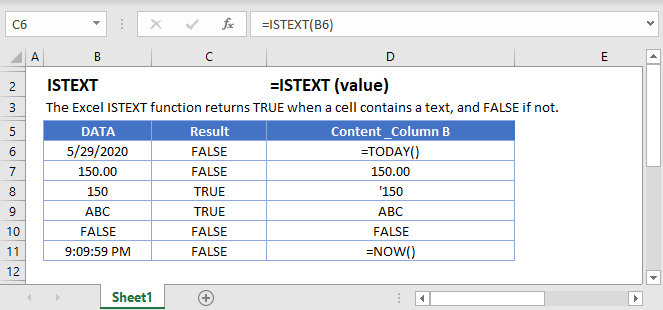## ISTEXT Function Description:

The ISTEXT Function Test if cell is text. Returns TRUE or FALSE.

To use the ISTEXT Excel Worksheet Function, select a cell and type: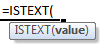(Notice how the formula inputs appear)

### ISTEXT Function Syntax and Inputs:

`=ISTEXT(VALUE)`

value – The test value

AutoMacro - VBA Code Generator

## How to use the ISTEXT Function

The ISTEXT Function tests if the cell contents are text. If the cell contains text it returns TRUE, otherwise it returns FALSE.

`=ISTEXT(A2)`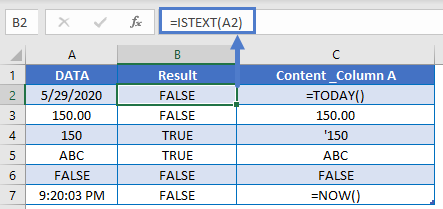### Numbers Stored as Text

When working with Excel, you might encounter numbers stored as text. Excel treats these numbers stored as text as text and not numbers, which can present problems for your formulas. One option is to use the VALUE Function to convert a number stored as text to a number.

Instead, however, you might simply want to test if a cell value contains text. Notice how the ISTEXT function returns TRUE if it encounters a number stored as text.

`=ISTEXT(A2)`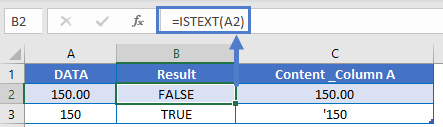### If Is Text

Usually, after performing a logical test, you will want to do something based on the result of that test. The IF Function can be used to perform different actions if a statement is evaluated as TRUE or FALSE. Let’s use it with the ISTEXT Function.

`=IF(ISTEXT(A2),"text", "not text")`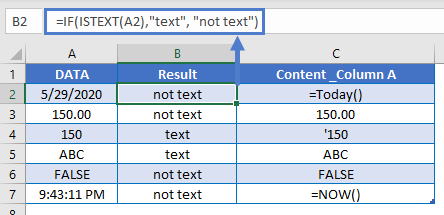### Validate Cell Input

One use of the ISTEXT Function is to validate the contents of a cell. For example, you might have an input form that requires a text value. With the ISTEXT Function you can test if the user entered a text value, alerting them if they did not.

`=IF(B2="","",IF(ISTEXT(B2),"","Invalid Entry"))`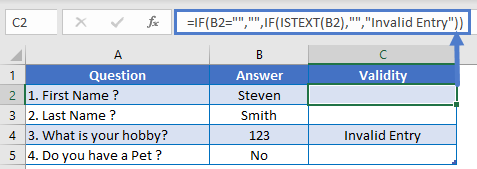### Data Validation – Force Text Entry

The above example was “weak” data validation; the user can ignore the warning message. Instead, we can use ISTEXT within Data Validation to disallow non-text values.

`=ISTEXT(B2:B5)`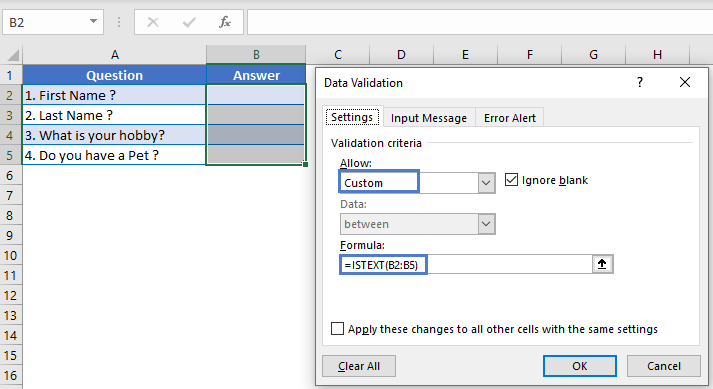<<<Note Steve – Please check this wording – Once set it not allowing input NONTEXT for this set area.>>>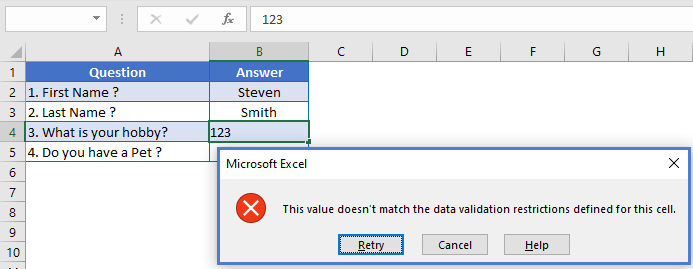### Test if Any Cell in Range is Text

To test if any cell in a range of cells is text, we can use the ISTEXT Function along with the SUMPRODUCT Function.

`=IF(SUMPRODUCT(--ISTEXT(A2:C2))>0, "Yes", "No")`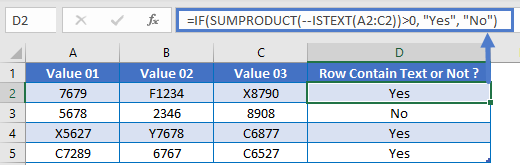Follow the link above to learn how this function works.

### Highlight Cells That Contain Text

One last thing you can do with the ISTEXT Function is highlight cells that contain text. We can do this by creating Conditional Formatting based on a rule.

1. Select all the cells that you want to check
2. Go to Home Ribbon > Conditional Formatting > New Rule
3. Select ‘Use a formula to determine which cells to format
4. Enter the formula =ISTEXT(A2)
5. Click the Format button and choose your desired formatting
6. Click OK twice to quit and save.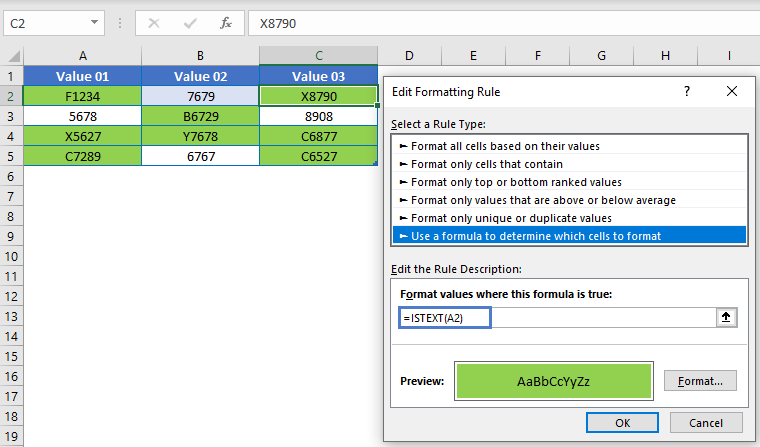### Other Logical Functions

Excel / Google Sheets contain many other logical functions to perform other logical tests. Here is a list:

IF / IS Functions
iferror
iserror
isna
iserr
isblank
isnumber
istext
isnontext
isformula
islogical
isref
iseven
isodd

## ISTEXT in Google Sheets

The ISTEXT Function works exactly the same in Google Sheets as in Excel: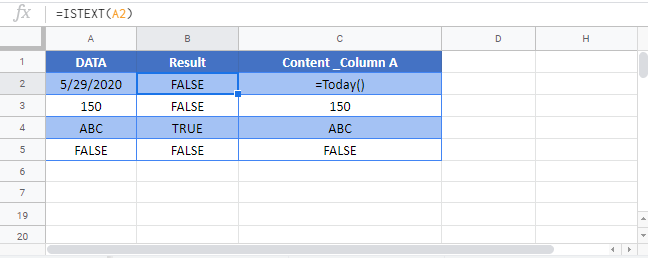## ISTEXT Examples in VBA

You can also use the ISTEXT function in VBA. Type:
`application.worksheetfunction.istext(value)`

Executing the following VBA statements

```Range("C2") = Application.WorksheetFunction.IsText(Range("B2"))
Range("C3") = Application.WorksheetFunction.IsText(Range("B3"))
Range("C4") = Application.WorksheetFunction.IsText(Range("B4"))
Range("C5") = Application.WorksheetFunction.IsText(Range("B5"))```

will produce the following results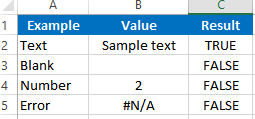For the function arguments (value, etc.), you can either enter them directly into the function, or define variables to use instead.

Return to the List of all Functions in Excel

## Excel Practice Worksheet

Practice Excel functions and formulas with our 100% free practice worksheets!

• Automatically Graded Exercises
• Learn Excel, Inside Excel!